In my previous post, I looked at the map Δ that takes a column vector to a diagonal matrix. I even drew a commutative diagram, which foreshadows a little category theory.

Suppose you have a function f of a real or complex variable. To an R programmer, if x is a vector, it’s obvious that f(x) means to apply f to every component of a vector. Python (NumPy) works the same way, and calls this broadcasting. To a mathematician, this looks odd. What does the logarithm of a vector, for example, even mean?

As in the previous post, we can use Δ to formalize things. We said that Δ has some nice properties, and in fact we will show it is a functor.

To have a functor, we have to have categories. (Historically, functors came first; categories were defined in order to define functors.) We will define C to be the category of column vectors and M the category of square matrices as before. Or rather, we should say the objects of C are column vectors and the objects of M are square matrices.

Categories need morphisms, functions between objects . We define the morphisms on C to be analytic functions applied componentwise. So, for example, if

z = [1, 2, -3],

then

tan(z) = [tan(1), tan(2), tan(-3)].

The morphisms on M will be analytic functions on square matrices, not applied componentwise but applied by power series. That is, given an analytic function f, we define f of a square matrix X as the result of sticking the matrix X into the power series for f. For an example, see What is the cosine of a matrix?

We said that Δ is a functor. It takes column vectors and turns them into square matrices by putting their contents along the diagonal of a matrix. We gave the example in the previous post that [4, i, π] would be mapped to the matrix with these elements on the diagonal, i.e.That says what Δ does on objects, but what does it do on morphisms? It takes an analytic function that was applied componentwise to column vectors, and turns it into a function that is applied via its power series to square matrices. That is, starting with a functionwe define the morphism f on C byand the morphism Δ f on M bywhere Z is a square matrix.

We can apply f to a column vector, and then apply Δ to turn the resulting vector into a diagonal matrix, or we could apply Δ to turn the vector into a diagonal matrix first, and then apply f (technically,  Δf). That is, the follow diagram commutes:## Python example

Applying an analytic function to a diagonal matrix gives the same result as simply applying the function to the elements of the diagonal. But for more general square matrices, this is not the case. We will illustrate this with some Python code.

    import numpy as np
from scipy.linalg import funm

d = np.array([1, 2])
D = np.diag(d)
M = np.array([[1, np.pi], [2, 0]])


Now let’s look at some output.

    >>> np.sin(d)
array([0.84147098, 0.90929743])

>>> np.sin(D)
array([[0.84147098, 0.        ],
[0.        , 0.90929743]])

>>> funm(D, np.sin)
array([[0.84147098, 0.        ],
[0.        , 0.90929743]])


So if we take the sine of d and turn the result into a matrix, we get the same thing as if we turn d into a matrix D and then take the sine of D, either componentwise or as an analytic function (with funm, function of a matrix).

Now let’s look at a general, non-diagonal matrix.

    >>> np.sin(M)
array([[0.84147099, 0],
[0.90929743, 0]])

>>> funm(D, np.sin)
array([[0.84147098, 0.        ],
[0.        , 0.90929743]])


Note that the elements in the bottom row are in opposite positions in the two examples.

 OK, morphisms are not necessarily functions, but in practice they usually are.

# Python and the Tell-Tale Heart

I was browsing through SciPy documentation this evening and ran across a function in scipy.misc called electrocardiogram. What?!

It’s an actual electrocardiogram, sampled at 360 Hz. Presumably it’s included as convenient example data. Here’s a plot of the first five seconds.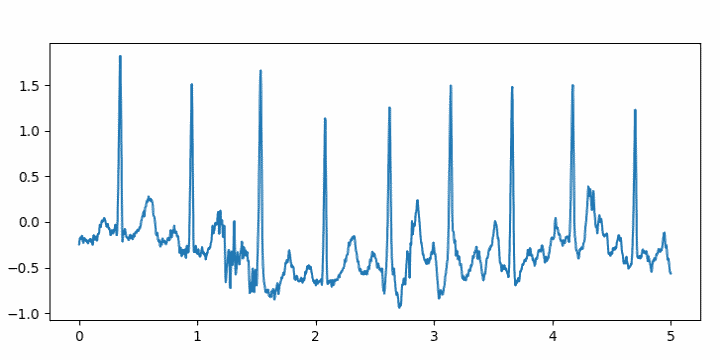I wrote a little code using it to turn the ECG into an audio file.

from numpy import int16, iinfo
from scipy.io.wavfile import write
from scipy.misc import electrocardiogram

def to_integer(signal):
# Take samples in [-1, 1] then scale to 16-bit integers
m = iinfo(int16).max
M = max(abs(signal))
return int16(signal*m/M)

ecg = electrocardiogram()
write("heartbeat.wav", 360, to_integer(ecg))


I had to turn the volume way up to hear it, and that made me think of Edgar Allan Poe’s story The Tell-Tale Heart.

I may be doing something wrong. According to the documentation for the write function, I shouldn’t need to convert the signal to integers. I should just be able to leave the signal as floating point and normalize it to [-1, 1] by dividing by the largest absolute value in the signal. But when I do that, the output file will not play.

# Stable and unstable recurrence relationsThe previous post looked at computing recurrence relations. That post ends with a warning that recursive evaluations may nor may not be numerically stable. This post will give examples that illustrate stability and instability.

There are two kinds of Bessel functions, denoted J and Y. These are called Bessel functions of the first and second kinds respectively. These functions carry a subscript n denoting their order. Both kinds of Bessel functions satisfy the same recurrence relation:

fn+1 – (2n/x) fn + fn-1 = 0

where f is J or Y.

If you apply the recurrence relation in the increasing direction, it is unstable for J but stable for Y.

If you apply the recurrence relation in the opposite direction, it is stable for J and unstable for Y.

We will illustrate the above claims using the following Python code. Since both kinds of Bessel function satisfy the same recurrence, we pass the Bessel function in as a function argument. SciPy implements Bessel functions of the first kind as jv and Bessel functions of the second kind as yv. 

    from scipy import exp, pi, zeros
from scipy.special import jv, yv

def report(k, computed, exact):
print(k, computed, exact, abs(computed - exact)/exact)

def bessel_up(x, n, f):
a, b = f(0, x), f(1, x)
for k in range(2, n+1):
a, b = b, 2*(k-1)*b/x - a
report(k, b, f(k,x))

def bessel_down(x, n, f):
a, b = f(n,x), f(n-1,x)
for k in range(n-2, -1, -1):
a, b = b, 2*(k+1)*b/x - a
report(k, b, f(k,x))


We try this out as follows:

    bessel_up(1, 20, jv)
bessel_down(1, 20, jv)
bessel_up(1, 20, yv)
bessel_down(1, 20, yv)


When we compute Jn(1) using bessel_up, the relative error starts out small and grows to about 1% when n = 9. The relative error increases rapidly from there. When n = 10, the relative error is 356%.

For n = 20, the recurrence gives a value of 316894.36 while the true value is 3.87e-25, i.e. the computed value is 30 orders of magnitude larger than the correct value!

When we use bessel_down, the results are correct to full precision.

Next we compute Yn(1) using bessel_up the results are correct to full precision.

When we compute Yn(1) using bessel_down, the results are about as bad as computing Jn(1) using bessel_up. We compute Y0(1) as 0 5.7e+27 while the correct value is roughly 0.088.

There are functions, such as Legendre polynomials, whose recurrence relations are stable in either direction, at least for some range of inputs. But it would be naive to assume that a recurrence is stable without some exploration.

## Miller’s algorithm

There is a trick for using the downward recurrence for Bessel functions known as Miller’s algorithm. It sounds crazy at first: Assume JN(x) = 1 and JN+1(x) = 0 for some large N, and run the recurrence downward.

Since we don’t know JN(x) our results be off by some constant proportion. But there’s a way to find out what that proportionality constant is using the relation described here.We add up out computed values for the terms on the right side, then divide by the sum to normalize our estimates. Miller’s recurrence algorithm applies more generally to other recurrences where the downward recurrence is stable and there exists a normalization identity analogous to the one for Bessel functions.

The following code lets us experiment with Miller’s algorithm.

    def miller(x, N):
j = zeros(N) # array to store values

a, b = 0, 1
for k in range(N-1, -1, -1):
a, b = b, 2*(k+1)*b/x - a
j[k] = b

norm = j + sum(2*j[k] for k in range(2,N,2))
j /= norm

for k in range(N-1, -1, -1):
expected, computed = j[k], jv(k,x)
report(k, j[k], jv(k,x))


When we call miller(pi, 20) we see that Miller’s method computes Jn(π) accurately. The error starts out moderately small and decreases until the results are accurate to floating point precision.

    |----+------------|
|  k | rel. error |
|----+------------|
| 19 |   3.91e-07 |
| 17 |   2.35e-09 |
| 16 |   2.17e-11 |
| 15 |   2.23e-13 |
| 14 |   3.51e-15 |
|----+------------|


For smaller k the relative error is also around 10-15, i.e. essentially full precision.

 Why do the SciPy names end in “v”? The order of a Bessel function does not have to be an integer. It could be any real number, and the customary mathematical notation is to use a Greek letter ν (nu) as a subscript rather than n as a reminder that the subscript might not represent an integer. Since a Greek ν looks similar to an English v, SciPy uses v as a sort of approximation of ν.

# Area of sinc and jinc function lobes

Someone left a comment this morning on my blog post on sinc and jinc integrals regarding the area of the lobes.

It would be nice to have the values of integrals of each lobe, i.e. integrals between 0 and multiples of pi. Anyone knows of such a table?

This post will include Python code to address that question. (Update: added asymptotic approximation. See below.)

First, let me back up and explain the context. The sinc function is defined as 

sinc(x) = sin(x) / x

and the jinc function is defined analogously as

jinc(x) = J1(x) / x,

substituting the Bessel function J1 for the sine function. You could think of Bessel functions as analogs of sines and cosines. Bessel functions often come up when vibrations are described in polar coordinates, just as sines and cosines come up when using rectangular coordinates.

Here’s a plot of the sinc and jinc functions:The lobes are the regions between crossings of the x-axis. For the sinc function, the lobe in the middle runs from -π to π, and for n > 0 the nth lobe runs from nπ to (n+1)π. The zeros of Bessel functions are not uniformly spaced like the zeros of the sine function, but they come up in application frequently and so it’s easy to find software to compute their locations.

First of all we’ll need some imports.

    from scipy import sin, pi
from scipy.special import jn, jn_zeros


The sinc and jinc functions are continuous at zero, but the computer doesn’t know that . To prevent division by zero, we return the limiting value of each function for very small arguments.

    def sinc(x):
return 1 if abs(x) < 1e-8 else sin(x)/x

def jinc(x):
return 0.5 if abs(x) < 1e-8 else jn(1,x)/x


You can show via Taylor series that these functions are exact to the limits of floating point precision for |x| < 10-8.

Here’s code to compute the area of the sinc lobes.

    def sinc_lobe_area(n):
n = abs(n)
integral, info = quad(sinc, n*pi, (n+1)*pi)
return 2*integral if n == 0 else integral


The corresponding code for the jinc function is a little more complicated because we need to compute the zeros for the Bessel function J1. Our solution is a little clunky because we have an upper bound N on the lobe number. Ideally we’d work out an asymptotic value for the lobe area and compute zeros up to the point where the asymptotic approximation became sufficiently accurate, and switch over to the asymptotic formula for sufficiently large n.

    def jinc_lobe_area(n):
n = abs(n)
assert(n < N)
integral, info = quad(jinc, jzeros[n-1], jzeros[n])
return 2*integral if n == 0 else integral


Note that the 0th element of the array returned by jn_zeros is the first positive zero of J1; it doesn’t include the zero at the origin.

For both sinc and jinc, the even numbered lobes have positive area and the odd numbered lobes have negative area. Here’s a plot of the absolute values of the lobe areas.## Asymptotic results

We can approximate the area of the nth lobe of the sinc function by using a midpoint approximation for 1/x. It works out that the area is asymptotically equal toWe can do a similar calculation for the area of the nth jinc lobe, starting with the asymptotic approximation for jinc given here. We find that the area of the nth lobe of the jinc function is asymptotically equal toTo get an idea of the accuracy of the asymptotic approximations, here are the results for n=100.

    sinc area:      0.00633455
asymptotic:     0.00633452
absolute error: 2.97e-8
relative error: 4.69e-6

jinc area:      0.000283391
asymptotic:     0.000283385
absolute error: 5.66e-9
relative error: 2.00e-5


## More signal processing posts

 Some authors define sinc(x) as sin(πx)/πx. Both definitions are common.

 Scipy has a sinc function in scipy.special, defined as sin(πx)/πx, but it doesn’t have a jinc function.

# Calculating the period of Van der Pol oscillators

A few days ago I wrote about how to solve differential equations with SciPy’s ivp_solve function using Van der Pol’s equation as the example. Van der Pol’s equation isThe parameter μ controls the amount of nonlinear damping. For any initial condition, the solution approach a periodic solution. The limiting periodic function does not depend on the initial condition  but does depend on μ. Here are the plots for μ  = 0, 1, and 2 from the previous post.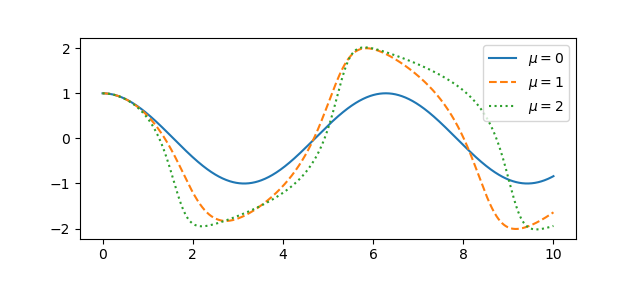A couple questions come to mind. First, how quickly do the solutions become periodic? Second, how does the period depend on μ? To address these questions, we’ll use an optional argument to ivp_solve we didn’t need in the earlier post.

## Using events in ivp_solve

For ivp_solve an event is a function of the time t and the solution y whose roots the solver will report. To determine the period, we’ll look at where the solution is zero; our event function is trivial since we want to find the roots of the solution itself.

Recall from the earlier post that we cast our second order ODE as a pair of first order ODEs, and so our solution is a vector, the function x and its derivative. So to find roots of the solution, we look at what the solver sees as the first component of the solver. So here’s our event function:

    def root(t, y): return y

Let’s set μ = 2 and find the zeros of the solution over the interval [0, 40], starting from the initial condition x(0) = 1, x‘(0) = 0.

    mu = 2
sol = solve_ivp(vdp, [0, 40], [1, 0], events=root)
zeros = sol.t_events


Here we reuse the vdp function from the previous post about the Van der Pol oscillator.

To estimate the period of the limit cycle we look at the spacing between zeros, and how that spacing is changing.

    spacing = zeros[1:] - zeros[:-1]
deltas = spacing[1:] - spacing[:-1]


If we plot the deltas we see that the zero spacings quickly approach a constant value. Zero crossings are half periods, so the period of the limit cycle is twice the limiting spacing between zeros.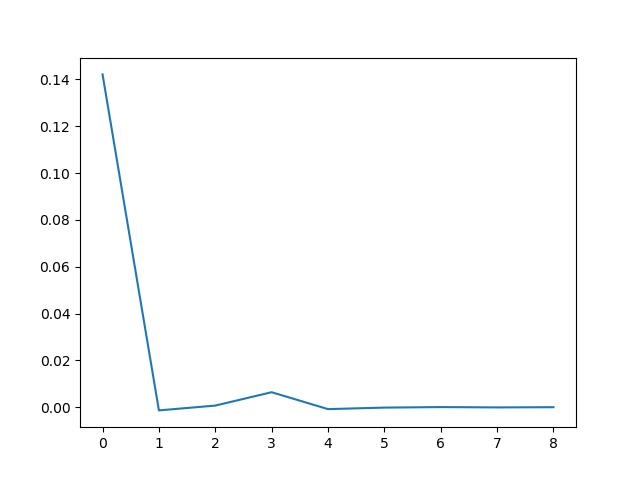## Theoretical results

If μ = 0 the Van der Pol oscillator reduces to a simple harmonic oscillator and the period is 2π. As μ increases, the period increases.

For relatively small μ we can calculate the period as above, but as μ increases this becomes more difficult numerically . But one can easily show that the period is asymptotically

T ~ (3 – 2 log 2) μ

as μ goes to infinity. A more refined estimate due to Mary Cartwright is

T ~ (3 – 2 log 2) μ + 2π/μ1/3

for large μ.

## More VdP posts

 There is a trivial solution, x = 0, corresponding to the initial conditions x(0) = x‘(0) = 0. Otherwise, every set of initial conditions leads to a solution that converges to the periodic attractor.

 To see why large values of μ are a problem numerically, here’s a plot of a solution for μ = 100.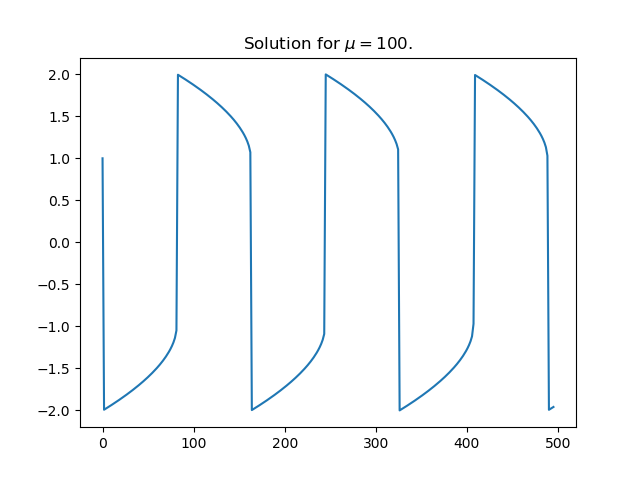The solution is differentiable everywhere, but the derivative changes so abruptly at the maxima and minima that it is discontinuous for practical purposes.

# Solving Van der Pol equation with ivp_solve

Van der Pol’s differential equation isThe equation describes a system with nonlinear damping, the degree of nonlinearity given by μ. If μ = 0 the system is linear and undamped, but as μ increases the strength of the nonlinearity increases. We will plot the phase portrait for the solution to Van der Pol’s equation in Python using SciPy’s new ODE solver ivp_solve.

The function ivp_solve does not solve second-order systems of equations directly. It solves systems of first-order equations, but a second-order differential equation can be recast as a pair of first-order equations by introducing the first derivative as a new variable.Since y is the derivative of x, the phase portrait is just the plot of (x, y).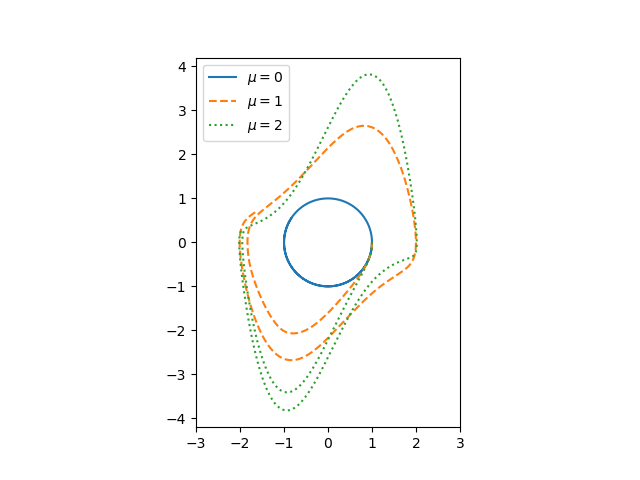If μ = 0, we have a simple harmonic oscillator and the phase portrait is simply a circle. For larger values of μ the solutions enter limiting cycles, but the cycles are more complicated than just circles. These limiting cycles are periodic attractors: every non-trivial solution converges to the limit cycle.

Here’s the Python code that made the plot.

from scipy import linspace
from scipy.integrate import solve_ivp
import matplotlib.pyplot as plt

def vdp(t, z):
x, y = z
return [y, mu*(1 - x**2)*y - x]

a, b = 0, 10

mus = [0, 1, 2]
styles = ["-", "--", ":"]
t = linspace(a, b, 500)

for mu, style in zip(mus, styles):
sol = solve_ivp(vdp, [a, b], [1, 0], t_eval=t)
plt.plot(sol.y, sol.y, style)

# make a little extra horizontal room for legend
plt.xlim([-3,3])
plt.legend([f"$\mu={m}$" for m in mus])
plt.axes().set_aspect(1)


To plot the solutions as a function of time, rather than plotting phase portraits, change the line

    plt.plot(sol.y, sol.y, style)

to

    plt.plot(sol.t, sol.y, style)

and comment out the line setting xlim This gives the following plot.# Illustrating Cayley-Hamilton with Python

If you take a square matrix M, subtract x from the elements on the diagonal, and take the determinant, you get a polynomial in x called the characteristic polynomial of M. For example, letThenThe characteristic equation is the equation that sets the characteristic polynomial to zero. The roots of this polynomial are eigenvalues of the matrix.

The Cayley-Hamilton theorem says that if you take the original matrix and stick it into the polynomial, you’ll get the zero matrix.In brief, a matrix satisfies its own characteristic equation. Note that for this to hold we interpret constants, like 12 and 0, as corresponding multiples of the identity matrix.

You could verify the Cayley-Hamilton theorem in Python using scipy.linalg.funm to compute a polynomial function of a matrix.

>>> from scipy import array
>>> from scipy.linalg import funm
>>> m = array([[5, -2], [1, 2]])
>>> funm(m, lambda x: x**2 - 7*x + 12)


This returns a zero matrix.

I imagine funm is factoring M into something like PDP-1 where D is a diagonal matrix. Then

f(M) = P f(D) P-1.

This is because f can be applied to a diagonal matrix by simply applying f to each diagonal entry independently. You could use this to prove the Cayley-Hamilton theorem for diagonalizable matrices.

# Data Science and Star Science

I recently got a review copy of Statistics, Data Mining, and Machine Learning in Astronomy. I’m sure the book is especially useful to astronomers, but those of us who are not astronomers could use it as a survey of data analysis techniques, especially using Python tools, where all the examples happen to come from astronomy. It covers a lot of ground and is pleasant to read.# Bessel function crossings

The previous post looked at the angles that graphs make when they cross. For example, sin(x) and cos(x) always cross with the same angle. The same holds for sin(kx) and cos(kx) since the k simply rescales the x-axis.

The post ended with wondering about functions analogous to sine and cosine, such as Bessel functions. This post will look at that question in more detail. Specifically we’ll look at the functions Jν and Yν.

Because these two Bessel functions satisfy the same second order linear homogeneous differential equation, the Strum separation theorem says that their zeros are interlaced: between each pair of consecutive zeros of Jν is exactly one zero of Yν, and between each pair of consecutive zeros of Yν there is exactly one zero of Jν.In the following Python code, we find zeros of Jν, then look in between for places where Jν and Yν cross. Next we find the angle the two curves make at each intersection and plot the angles.

    from scipy.special import jn_zeros, jv, yv
from scipy.optimize import bisect
from numpy import empty, linspace, arccos
import matplotlib.pyplot as plt

n = 3 # bessel function order
N = 100 # number of zeros

z = jn_zeros(n, N) # Zeros of J_n
crossings = empty(N-1)

f = lambda x: jv(n,x) - yv(n,x)
for i in range(N-1):
crossings[i] = bisect(f, z[i], z[i+1])

def angle(n, x):
# Derivatives of J_nu and Y_nu
dj = 0.5*(jv(n-1,x) - jv(n+1,x))
dy = 0.5*(yv(n-1,x) - yv(n+1,x))

top = 1 + dj*dy
bottom = ((1 + dj**2)*(1 + dy**2))**0.5
return arccos(top/bottom)

y = angle(n, crossings)
plt.plot(y)
plt.xlabel("Crossing number")
plt.show()This quadratic behavior is what we should expect from the asymptotics of Jν and Yν: For large arguments they act like shifted and rescaled versions of sin(x)/√x. So if we looked at √xJν and √xYν rather than Jν and Yν we’d expect the angles to reach some positive asymptote, and they do, as shown below.# Clearing up the confusion around Jacobi functions

The Jacobi elliptic functions sn and cn are analogous to the trigonometric functions sine and cosine. The come up in applications such as nonlinear oscillations and conformal mapping. Unfortunately there are multiple conventions for defining these functions. The purpose of this post is to clear up the confusion around these different conventions.The image above is a plot of the function sn .

## Modulus, parameter, and modular angle

Jacobi functions take two inputs. We typically think of a Jacobi function as being a function of its first input, the second input being fixed. This second input is a “dial” you can turn that changes their behavior.

There are several ways to specify this dial. I started with the word “dial” rather than “parameter” because in this context parameter takes on a technical meaning, one way of describing the dial. In addition to the “parameter,” you could describe a Jacobi function in terms of its modulus or modular angle. This post will be a Rosetta Stone of sorts, showing how each of these ways of describing a Jacobi elliptic function are related.

The parameter m is a real number in [0, 1]. The complementary parameter is m‘ = 1 – m. Abramowitz and Stegun, for example, write the Jacobi functions sn and cn as sn(um) and cn(um). They also use m1 = rather than m‘ to denote the complementary parameter.

The modulus k is the square root of m. It would be easier to remember if m stood for modulus, but that’s not conventional. Instead, m is for parameter and k is for modulus. The complementary modulus k‘ is the square root of the complementary parameter.

The modular angle α is defined by m = sin² α.

Note that as the parameter m goes to zero, so does the modulus k and the modular angle α. As any one of these three goes to zero, the Jacobi functions sn and cn converge to their counterparts sine and cosine. So whether your dial is the parameter, modulus, or modular angle, sn converges to sine and cn converges to cosine as you turn the dial toward zero.

As the parameter m goes to 1, so does the modulus k, but the modular angle α goes to π/2. So if your dial is the parameter or the modulus, it goes to 1. But if you think of your dial as modular angle, it goes to π/2. In either case, as you turn the dial to the right as far as it will go, sn converges to hyperbolic secant, and cn converges to the constant function 1.

## Quarter periods

In addition to parameter, modulus, and modular angle, you’ll also see Jacobi function described in terms of K and K‘. These are called the quarter periods for good reason. The functions sn and cn have period 4K as you move along the real axis, or move horizontally anywhere in the complex plane. They also have period 4iK‘. That is, the functions repeat when you move a distance 4K‘ vertically .

The quarter periods are a function of the modulus. The quarter period K along the real axis isand the quarter period K‘ along the imaginary axis is given by K‘(m) = K(m‘) = K(1 – m).

The function K(m) is known as “the complete elliptic integral of the first kind.”

## Amplitude

So far we’ve focused on the second input to the Jacobi functions, and three conventions for specifying it.

There are two conventions for specifying the first argument, written either as φ or as u. These are related byThe angle φ is called the amplitude. (Yes, it’s an angle, but it’s called an amplitude.)

When we said above that the Jacobi functions had period 4K, this was in terms of the variable u. Note that when φ = π/2, uK.

## Jacobi elliptic functions in Mathematica

Mathematica uses the u convention for the first argument and the parameter convention for the second argument.

The Mathematica function JacobiSN[u, m] computes the function sn with argument u and parameter m. In the notation of A&S, sn(um).

Similarly, JacobiCN[u, m] computes the function cn with argument u and parameter m. In the notation of A&S, cn(um).

We haven’t talked about the Jacobi function dn up to this point, but it is implemented in Mathematica as JacobiDN[u, m].

The function that solves for the amplitude φ as a function of u is JacobiAmplitude[um m].

The function that computes the quarter period K from the parameter m is EllipticK[m].

## Jacobi elliptic functions in Python

The SciPy library has one Python function that computes four mathematical functions at once. The function scipy.special.ellipj takes two arguments, u and m, just like Mathematica, and returns sn(um), cn(um), dn(um), and the amplitude φ(um).

The function K(m) is implemented in Python as scipy.special.ellipk.

## Related posts

 The plot was made using JacobiSN[0.5, z] and the function ComplexPlot described here.

 Strictly speaking, 4iK‘ is a period. It’s the smallest vertical period for cn, but 2iK‘ is the smallest vertical period for sn.